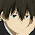Theme images by Storman. Powered by Blogger.

Current Sourcing and Current Sinking in TTL

The difference between current sourcing and current sinking is as follows:

We know that a logic gate will always remain either in the 0 state or in the 1 state. For a TTL gate, when the gate is in the 1 state, its output (transistor T4) is at +VCC. This high output voltage of the gate can drive many other gates connected in parallel to it by supplying the individual currents required to drive them. This operation is called current sourcing, since the output transistor is capable of acting as a current source. Figure 3.13 shows the current-sourcing operation. It is found that in the logic-1 state, a TTL NAND gate can source (deliver) up to 40 mA of current, as shown in the figure.

The sinking operation is illustrated in Fig. 3.14. In the current-sinking process that occurs in the logic-0 state, the output transistor acts as a sink for other gates driven by it. The maximum value of the sinking current of a TTL NAND gate is 16 mA.

Fan-in Capability of IC Gates

Fan-in represents the maximum number of inputs that can be connected to an 1C gate. In effect, it represents the maximum number of input pins in a given logic-gate chip. For example, 7400 IC chip represents a two-input TTL NAND gate. Therefore, we say that the fan-in of 7400 is 2. Similarly, 7430 IC chip has 8 inputs, and hence its fan-in is 8.

Fan-out Capability of IC Gates

Fan-out represents the number of gates that a given IC can drive. To calculate the fan-out capability of a TTL NAND gate, we proceed as follows:

It has been computed that the sinking current of a TTL gate is 16 mA. However, the input current required by each driven gate is 1.6 mA in the sinking mode. Thus the 16-mA current of a TTL gate can sink up to a maximum of ten 1.6-mA TTL gates. Therefore:

Fan-out of a typical TTL gate = 16 mA/1.6 mA = 10

In the sourcing mode also, this same value remains applicable because typical sourcing current is 40 mA for the driven gate. Therefore:

Fan-out of a typical TTL gate = 40 mA/4 mA = 10

1 on: "Current Sourcing and Current Sinking in TTL"
1.Thanks buddy. This helped.